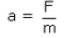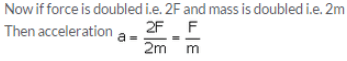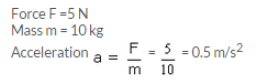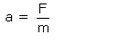Lakhmir Singh & Manjit Kaur: Force and Law of Motion, Solutions- 2

# Lakhmir Singh & Manjit Kaur: Force and Law of Motion, Solutions- 2 - Science Class 9

Page No - 56

Question 26:
Which of the two, balanced forces or unbalanced forces, can change the shape of an object ? Give an example to illustrate your answer.
Solution :
Balanced forces can change the shape of the object. For example, when a balloon is pressed between hands, then balanced forces (equal and opposite forces) act on the balloon due to which the shape of the balloon changes.

Question 27:
Describe the term ‘inertia’ with respect to motion.
Solution :
Inertia of motion is the property of a body due to which it resists a change in its state of uniform motion. For eg., if there is no air resistance and no friction to oppose the motion of a moving bicycle, it will go on moving forever.

Question 28:
State Newton’s first law of motion. Give two examples to illustrate Newton’s first law of motion.
Solution :
Newton’s first law of motion states that a body at rest will remain at rest, and a body in motion will continue in motion in a straight line with a uniform speed unless it is compelled by an external force to change its state of rest or of uniform motion. For example, a book lying on a table remains on the table unless we lift it with the force of our hands. And, on a frictionless surface, a moving car continues to be in the state of motion until brakes are applied on it.

Question 29:
On what factor does the inertia of a body depend ? Which has more inertia, a cricket ball or a rubber ball of the same size ?
Solution :
Inertia of a body depends on its mass. A cricket ball has more inertia than a rubber ball of the same size because it has more mass than the rubber ball.

Question 30:
Why do the passengers in a bus tend to fall backward when it starts suddenly ?
Solution :
When a bus starts suddenly, its passengers tend to fall backwards because due to their inertia, the passengers tend to remain in a state of rest even when the bus starts moving.

Question 31:
Explain why, a person travelling in a bus falls forward when the bus stops suddenly.
Solution :
When a bus stops suddenly, its passengers tend to fall forward because due to their inertia, the passengers tend to remain in a state of motion even though the bus has come to rest.

Question 32:
Give reason for the following :
When a hanging carpet is beaten with a stick, the dust particles start coming out of it.
Solution :
When a hanging carpet is beaten with a stick, the carpet moves to and fro with the force of the stick while the dust particles remain in their state of rest on account of their inertia and thus dust particles separate out from the carpet.

Question 33:
When a tree is shaken, its fruits and leaves fall down: Why ?
Solution :
When a tree is shaken, the tree moves to and fro while the fruits and leaves remain in their state of rest on account of their inertia and thus fruits and leaves separate from the tree and fall from the tree.

Question 34:
Explain why, it is dangerous to jump out of a moving bus.
Solution :
It is dangerous to jump out of a moving bus because the jumping man, who is moving with the high speed of the bus would tend to remain in state of motion due to inertia even on falling to the ground and get hurt due to resistance offered by the ground.

Question 35:
What is the momentum in kg.m/s of a 10 kg car travelling at (a) 5 m/s (b) 20 cm/s, and (c) 36 km/h ?
Solution :
Mass of car, m = 10 kg
Momentum = m x v
a) Velocity, v = 5 m/s Momentum = 10 x 5 = 50 kg.m/s
b) Velocity, v = 20 cm/s = 0.2 m/s Momentum = 10 x 0.2 = 2 kg.m/s
c) Velocity, v = 36km/hr = 10 m/s Momentum = 10 x 10 = 100 kg.m/s

Question 36:
(a) Define momentum of a body. On what factors does the momentum of a body depend ?
(b) Calculate the change in momentum of a body weighing 5 kg when its velocity decreases from 20 m/s to 0.20 m/s.
Solution :
a) Momentum is the physical quantity which is the measure of the quantity of motion of a moving body. It depends on mass and velocity of the body.
b) Mass of body = 5 kg
Velocity v1 = 20 m/s
Momentum p1 = 20 x 5 = 100 kg.m/s
Velocity v2 = 0.2 m/s
Momentum p2 = 5 x 0.2 = 1 kg.m/s
Change in momentum = p2– p1= 1-100 = -99 kg.m/s (Negative sign shows that momentum decreases)

Question 37:
(a) Define the term ‘force’.
(b) State the various effects of force.
Solution :
a) Force is an influence which tends to set a stationary body in motion or stop a moving body; or which tends to change the speed and direction of a moving body; or which tends to change the shape and size of a body.
b) Various effects of force are
i) A force can move a stationary body.
ii) A force can stop a moving body.
iii) A force can change the speed of a moving body.
iv) A force can change the direction of a moving body.
v) A force can change the shape and size of a body.

Question 38:
Give one example each where :
(a) a force moves a stationary body.
(b) a force stops a moving body.
(c) a force changes the speed of a moving body.
(d) a force changes the direction of a moving body.
(e) a force changes the shape (and size) of a body.
Solution :
a) Kicking a stationary football.
b) Applying brakes to a moving bicycle.
c) Pressing an accelerator to speed up a moving car.
d) A moving cricket ball hit by a bat.
e) Flattening of dough by a rolling pin to make chapatis.

Question 39:
(a) What do you understand by the terms “balanced forces” and “unbalanced forces” ? Explain with examples.
(b) What type of forces – balanced or unbalanced – act on a rubber ball when we press it between our hands ? What effect is produced in the ball ?
Solution :
a) If the resultant of all the forces acting on a body is zero, the forces are called balanced forces. These forces do not change the state of rest or of uniform motion of a body but can change the shape of the body. For example, when a balloon is pressed between hands, then balanced forces (equal and opposite forces) act on the balloon due to which the shape of the balloon changes.
If the resultant of all the forces acting on a body is not zero, the forces are called unbalanced forces. These forces change the state of rest or of uniform motion of a body. For eg., if we push a toy car lying on the ground, it starts moving due to the unbalanced force exerted by our hands.
b) When we press a rubber ball between our hands, balanced forces acts on it and hence its shape changes.

Question 40:
(a) What happens to the passengers travelling in a bus when the bus takes a sharp turn ? Give reasons for
(b) Why are road accidents at high speeds very much worse than road accidents at low speeds ?Solution :
a) When a bus takes a sharp turn, the passengers tend to fall sideways because of their inertia or their tendency to continue moving in a straight line.
b) Road accidents at high speeds are much worse than road accidents at low speeds because the momentum of vehicles at high speeds is very high and causes a lot of damage to the vehicles and injuries to the passengers during collision.

Page No - 57

Question 51:
A plastic ball and a clay ball of equal masses, travelling in the same direction with equal speeds, strike against a vertical wall. From which ball does the wall receive a greater amount of momentum ?
Solution :
The wall will receive equal momentum from both the balls because both balls have equal mass and velocity.

Question 52:
A moving bicycle comes to rest after sometime if we stop pedalling it. But Newton’s first law of motion says that a moving body should continue to move for ever, unless some external force acts on it. How do you explain the bicycle case ?
Solution :
In this case, the bicycle has been compelled to change its state of motion by the external force of air resistance and friction. If there were no air resistance and no friction to oppose the motion of the bicycle, then according to the first law of motion, the bicycle would go on moving forever.

Question 53:
A man throws a ball weighing 500 g vertically upwards with a speed of 10 m/s.

1. What will be its initial momentum ?
2. What would be its momentum at the highest point of its flight ?

Solution :
Mass of ball = 500 g = 0.5 kg
Initial velocity = 10 m/s

1. Initial momentum = 0.5 x 10 = 5 kg.m/s
2. Velocity at the highest point = 0 m/s

Momentum at the highest point = 0.5 x 0 = 0 kg.m/s

Question 54:
A car is moving on a level road. If the driver turns off the engine of the car, the car’s speed decreases gradually and ultimately it comes to a stop. A student says that two forces act on the car which bring it to a stop. What could these forces be ? Which of these two forces contributes more to slow down and stop the car ?
Solution :
The two forces acting on the car are force of friction and air resistance. Force of friction contributes more to slow down and stop the car.

Question 55:
There are two types of forces X and Y. The forces belonging to type X can produce motion in a stationary object but cannot change the shape of the object. On the other hand, forces belonging to type Y cannot produce motion in a stationary object but can change the shape of the object. What is the general name of the forces such as (a) X, and (b) Y ?
Solution :
a) X are unbalanced force.
b) Y are balanced forces.

Page No - 74

Question 1:
Which physical quantity corresponds to the rate of change of momentum ?
Solution :
Force corresponds to the rate of change of momentum.

Question 2:
State the relation between the momentum of a body and the force acting on it.
Solution :
The rate of change of momentum of a body is directly proportional to the applied force and takes place in the direction in which the force acts.

Question 3:
What is tire unit of force ?
Solution :
The SI unit of force is newton(N)

Question 4:
Define one newton force.
Solution :
A newton force is defined as that force which when acting on a body of mass of 1 kg produces an acceleration of 1m/s2 in it.

Question 5:
What is the relationship between force and acceleration ?
Solution :
Force acting on a body is directly proportional to the acceleration produced in the body.

F∝a

Question 6:
If the mass of a body and the force acting on it are both doubled, what happens to the acceleration ?

Solution :

Acceleration remains same sinceQuestion 7:
Name the physical quantity whose unit is ‘newton’.
Solution :
Newton is the SI unit of force.

Question 8:
Which physical principle is involved in the working of a jet aeroplane ?
Solution :
Jet airplanes work on the principle of conservation of momentum.

Question 9:
Name the principle on which a rocket works.
Solution :
Rockets work on the principle of conservation of momentum.

Question 10:
Is the following statement true or false :
A rocket can propel itself in a vacuum.

Solution :
True, because rocket does not require air for obtaining uplift or for burning its fuel.

Question 11:
What is the force which produces an acceleration of 1 m /s in a body of mass 1 kg ?
Solution :
Mass, m = 1 kg
Acceleration, a =
1m/s2
Force F = m x a = 1
x 1= 1N

Question 12:
Find the acceleration produced by a force of 5 N acting on a mass of 10 kg.
Solution :
Force F =5 N
Mass m = 10 kgQuestion 13:
A girl weighing 25 kg stands on the tloor. She exerts a downward force of 250 N on the floor. What force does the floor exert on her ?
Solution :
The force exerted by the floor on her = the downward force exerted by the girl =250 N
This is due to Newton’s third law of motion which states that to every action there is an equal and opposite reaction.Question 14:
Name the physical quantity which makes it easier to accelerate a small car than a large car.
Solution :
Less mass of the small car makes it easier to accelerate a small car than a large car because acceleration is inversely
proportional to mass of the car

Question 15:
Fill in the following blanks with suitable words :
(a) To every action, there is an………………….. and…………………… reaction
(b)  Momentum is a…………….. Its emit is……………………
(c)  Newton’s second law of motion can be written as Force = mass x …………………………… or Force = ……………….. of change of……………….
(d)  Forces in a Newton’s third law pair have equal………………… but act in opposite………………..
(e)  In collisions and explosions, the total ……………… remains constant, provided that no external……………………..

Solution :
a) Equal; opposite
b) Vector; kg.m/s
c) Acceleration; rate; momentum
d) Magnitude; directions
e) Momentum; force

Question 16:
Explain the meaning of the following equation :
F = m x a
where symbols have their usual meanings

Solution :
Force is directly proportional to the product of ‘mass’ of the body and the ‘acceleration’ produced in the body by the action of force.
F = m x a
where F is the force applied on the body
m is the mass of the body
a is the acceleration produced in the body

Question 17:
To take the boat away from the bank of a river, the boatman pushes the bank with an oar. Why ?
Solution :
To take boat away from the bank of a river, the boatman pushes the bank with the oar. The bank exerts an equal and opposite force on the boat which makes the boat move forward away from the bank.

Question 18:
Why does a gunman get a jerk on firing a bullet ?Solution :
Gunman gets a jerk on firing a bullet because when a bullet is fired from a gun, the force sending the bullet forward is equal to the force sending the gun backwards but due to high mass of the gun, it moves only a little distance backwards giving a jerk to the gunman.

Question 19:
If action is always equal to reaction, explain why a cart pulled by a horse can be moved.
Solution :
To make the cart move, the horse bends forward and pushes the ground with its feet. When the forward reaction to the backward push of the horse on the ground is greater than the opposing frictional forces of the wheels, the cart moves.

The document Lakhmir Singh & Manjit Kaur: Force and Law of Motion, Solutions- 2 | Science Class 9 is a part of the Class 9 Course Science Class 9.
All you need of Class 9 at this link: Class 9

## FAQs on Lakhmir Singh & Manjit Kaur: Force and Law of Motion, Solutions- 2 - Science Class 9

 1. What is the definition of force according to the Law of Motion?Ans. According to the Law of Motion, force is defined as the push or pull upon an object that results from its interaction with another object.
 2. How are Newton's laws of motion related to the article?Ans. Newton's laws of motion are the foundation of the article as it discusses the concepts of force and the laws governing the motion of objects. The article provides solutions related to the application of these laws.
 3. What are the three laws of motion stated by Newton?Ans. Newton's three laws of motion are: 1. The law of inertia - An object at rest will remain at rest, and an object in motion will continue to move in a straight line at a constant speed unless acted upon by an external force. 2. The law of acceleration - The acceleration of an object is directly proportional to the net force acting on it and inversely proportional to its mass. 3. The law of action and reaction - For every action, there is an equal and opposite reaction.
 4. How can the concept of force be applied in our daily lives?Ans. The concept of force can be applied in various aspects of our daily lives, such as: - Pushing or pulling objects. - Lifting or moving heavy objects. - Riding a bicycle or driving a car. - Playing sports like football or tennis, where force is used to kick or hit the ball. - Opening or closing doors, windows, or any other objects.
 5. What is the significance of understanding the force and laws of motion?Ans. Understanding force and the laws of motion is significant because: - It helps us understand how objects move and interact with each other. - It enables us to predict the behavior of objects in different scenarios. - It has practical applications in fields like engineering, physics, and sports. - It allows us to design and build structures, machines, and vehicles that function effectively. - It provides a foundation for advanced concepts in physics and other related sciences.

## Science Class 9

98 videos|369 docs|148 tests

## Science Class 9

98 videos|369 docs|148 tests
Signup to see your scores go up within 7 days! Learn & Practice with 1000+ FREE Notes, Videos & Tests.
10M+ students study on EduRev
Track your progress, build streaks, highlight & save important lessons and more!(Scan QR code)
Related Searches

,

,

,

,

,

,

,

,

,

,

,

,

,

,

,

,

,

,

,

,

,

,

,

,

;